# Colleges with the lowest SAT scores in Florida

Top 10 colleges in Florida with the lowest SAT scores
Looking for the colleges with the lowest SAT scores in Florida? Well you're in luck! We've compiled a national college database and have created a list of the top 10 universities with the lowest SAT scores in Florida below. If you are not a good test taker or worried about your test scores, this list is for you. These are the schools whose applicants had the lowest average SAT scores in Florida, which means that you can get into these colleges with a lower SAT score. We also include each college's ACT scores and acceptance rate so that you can see where you would have the easiest time getting in. Read on to find out more.

## Bethune-Cookman University SAT scores

The average SAT score for Bethune-Cookman University is 800.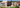The average SAT score of 800 breaks down into:

• SAT math: 390

The average ACT score for Bethune-Cookman University is 16 and their acceptance rate is 54.3%.

## Barry University SAT scores

The average SAT score for Barry University is 940.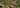The average SAT score of 940 breaks down into:

• SAT math: 510

The average ACT score for Barry University is 19 and their acceptance rate is 85.1%.

## Warner University SAT scores

The average SAT score for Warner University is 950.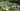The average SAT score of 950 breaks down into:

• SAT math: 480

The average ACT score for Warner University is 18 and their acceptance rate is 41%.

## Jacksonville University SAT scores

The average SAT score for Jacksonville University is 1010.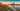The average SAT score of 1010 breaks down into:

• SAT math: 500

The average ACT score for Jacksonville University is 23 and their acceptance rate is 89.9%.

## Webber International University SAT scores

The average SAT score for Webber International University is 1010.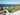The average SAT score of 1010 breaks down into:

• SAT math: 500

The average ACT score for Webber International University is 19 and their acceptance rate is 49%.

## Saint Leo University SAT scores

The average SAT score for Saint Leo University is 1024.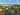The average SAT score of 1024 breaks down into:

• SAT math: 520

The average ACT score for Saint Leo University is 23 and their acceptance rate is 84.1%.

## Lynn University SAT scores

The average SAT score for Lynn University is 1048.The average SAT score of 1048 breaks down into:

• SAT math: 520

The average ACT score for Lynn University is 21 and their acceptance rate is 84.6%.

## Flagler College SAT scores

The average SAT score for Flagler College is 1060.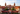The average SAT score of 1060 breaks down into:

• SAT math: 490

The average ACT score for Flagler College is 23 and their acceptance rate is 56.6%.

## Palm Beach Atlantic University SAT scores

The average SAT score for Palm Beach Atlantic University is 1110.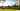The average SAT score of 1110 breaks down into:

• SAT math: 540

The average ACT score for Palm Beach Atlantic University is 23 and their acceptance rate is 95.3%.

## University of West Florida SAT scores

The average SAT score for University of West Florida is 1110.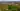The average SAT score of 1110 breaks down into:

• SAT math: 540